Page top# Temperature Controllers

These Controllers receive sensor signals and control heaters or other devices to maintain a preset temperature. They can also be used for humidity, pressure, and flowrate control. OMRON also provides temperature and humidity sensors.

 Introduction Features PrinciplesClassifications Engineering DataFurther InformationExplanation of Terms Troubleshooting
﻿

What Is a Temperature Controller?

A Temperature Controller is a device that is used to control a heater or other equipment by comparing a sensor signal with a set point and performing calculations according to the deviation between those values. Devices that can handle sensor signals other than for temperature, such as humidity, pressure, and flow rate, are called Controllers. Electronic controllers are specifically called Digital Controllers.

Temperature Control

Temperature Controllers control temperature so that the process value will be the same as the set point, but the response will differ due to the characteristics of the controlled object and the control method of the Temperature Controller. Typically, a response shown in Figure (2), where the set point is reached as quick as possible without overshooting, is required in a Temperature Controller. There are also cases such as the one shown in Figure (1), where a response quickly increases the temperature even if it overshoots is required, and the one shown in Figure (3), where a response slowly increases the temperature is required.

(1) Response where the process value settles on the set point while repeatedly overshooting and undershooting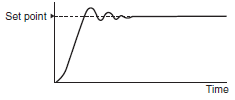(2) Proper response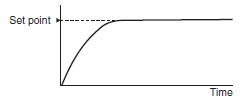(3) Response where the process value slowly reaches the set point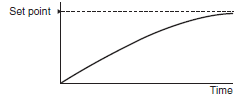Temperature Control Configuration Example

The following example describes the basic configuration for temperature control.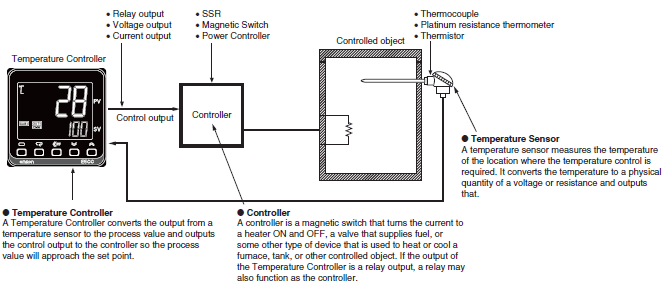Temperature Controller Principle

The following figure shows an example of a feedback control system used for temperature control.
The major parts of the feedback control system are built into the Temperature Controller. A feedback control system can be built and temperature can be controlled by combining a Temperature Controller with a controller and temperature sensor that are suitable for the controlled object.

Configuration of a Feedback Control System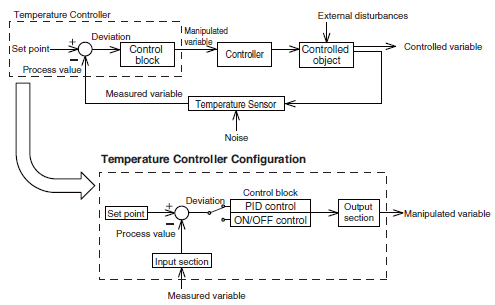Characteristics of the Controlled Object

Before selecting a Temperature Controller or temperature sensor, it is necessary to understand the thermal characteristics of the controlled object for proper temperature control.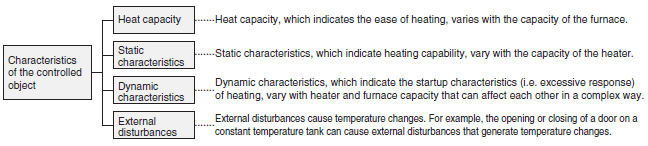Control Methods

[ON/OFF Control Action]

As shown in the graph below, if the process value is lower than the set point, the output will be turned ON and power will be supplied to the heater. If the process value is higher than the set point, the output will be turned OFF and power to the heater will be shut off. This control method, in which the output is turned ON and OFF based on the set point in order to keep the temperature constant, is called ON/OFF control action. With this action, the temperature is controlled using two values (i.e., 0% and 100% of the set point). Therefore, the operation is also called two-position control action.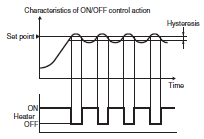[P Action (Proportional Control Action)]

P action (or proportional control action) is used to output a manipulated variable (control output variable) that is proportional to the deviation in order to decrease the deviation between the process value and set point. A proportional band is set centering on the set point, and the output is determined with the following rules.

A manipulated variable that is proportional to the deviation is output when the process value is within the proportional band.

A 100% manipulated variable is output when the process value is lower than the proportional band.

A 0% manipulated variable is output when the process value is higher than the proportional band.

Smoother control than the ON/OFF control action is possible because the output is gradually changed near the set point according to deviation. However, if the temperature is controlled with the proportional action alone, it will stabilize at a temperature that is off from the set point (offset).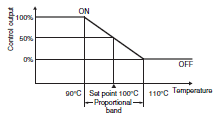Note: If a Temperature Controller with a temperature range of 0°C to 400°C has a 5% proportional band, the width of the proportional band will be converted into a temperature range of 20°C. In this case, a full output is kept turned ON until the process value reaches 90°C, and the output is OFF periodically when the process value exceeds 90°C, provided the set point is 100°C. When the process value is 100°C, there will be no difference in time between the ON period and the OFF period (i.e. the output is turned ON and OFF 50% of the time.)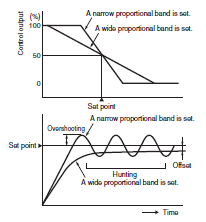[I Action (Integral Control Action)]

I action (or integral action) increases or decreases the manipulated variable according to the size and duration of the deviation.
The temperature will stabilize at a temperature off from the set point (offset) with only the proportional action, but the deviation with the passage of time will be decreased and the process value will be the same as the set point by combining the proportional and integral actions.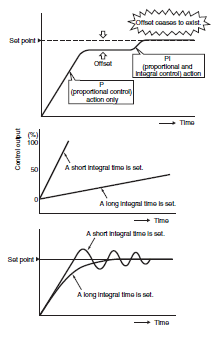[D Action (Derivative Control Action)]

D action (or derivative action) provides a manipulated variable in response to abrupt changes in the process value, due to factors such as an external disturbance, so that control will quickly return to the original status.The proportional and integral actions both corrects the control results, so the response to abrupt changes is delayed. The derivative action compensates for that drawback and provides a large manipulated variable for rapid external disturbances.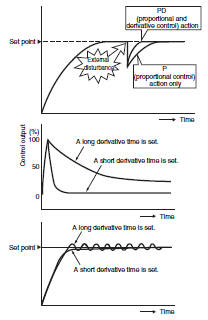[PID Control]

PID control is a combination of proportional, integral, and derivative control actions. The temperature is controlled smoothly here by proportional control action without hunting, automatic offset adjustment is made by integral control action, and quick response to an external disturbance is made possible by derivative control action.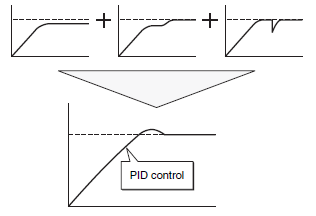[Two PID Control]

Conventional PID control uses a single control block to control the responses of the Temperature Controller to a set point and to external disturbances. Therefore, the response to the set point will oscillate due to overshooting if importance is placed on responding to external disturbances with the P and I parameters set to small values and the D parameter set to a large value in the control block. On the other hand, the Temperature Controller will not be able to respond to external disturbances quickly if importance is placed on responding to the set point (i.e., the P and I parameters are set to large values). This makes it impossible to satisfy both the types of response in this case.
Two PID control provides good response for both response to the set point and an external disturbance.

PID Control

(1)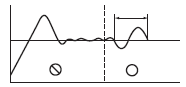Response to the set point will be slow if response to the external disturbance is improved.

(2)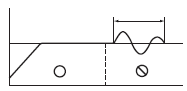Response to the external disturbance will be slow if response to the set point is improved.

Two PID Control

(3)Controls both the set point and the external disturbance response.

What Is a Temperature Sensor?

A Temperature Sensor measures the temperature of a location where the temperature control is required. It converts the temperature to a physical quantity of a voltage or resistance and outputs that.

Temperature Measurement Categories

There are two categories of temperature measurement, as described below.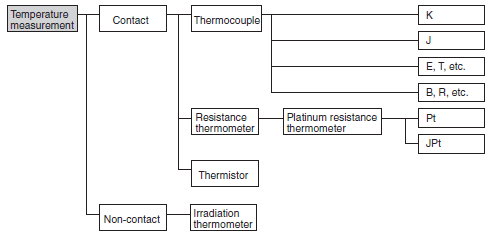Thermocouple

Principle

A thermocouple is a temperature sensor that uses a phenomenon (i.e., the Seebeck effect) that generates a thermoelectromotive force according to the temperature difference between the joint end and the open end of different types of metal that have been joined together at one end. The combination of metals with high and stable thermoelectromotive force is called a thermocouple.
Thermocouples are widely used in industry.

The Law of Intermediate Temperatures and the Law of Intermediate Metals

The size of the potential difference is determined by the two different materials of the metal wires and by the difference in temperature between the thermocouple junction (i.e., hot junction) and reference junction (i.e., cold junction). Any difference in temperature in between has no effect (Law of Intermediate Temperatures). There is also no effect if there are different types of metals in between as long as there is no difference in temperature (Law of Intermediate Metals).Thermocouple Types

Among thermocouples, types K, E, J, and T use base metals, and types B, R, and S use noble metals.
The type of thermocouple is chosen based on the measurement temperature, environment, and accuracy. In general, however, types K, J, and R are commonly used.

Characteristics of Thermocouple Potential Difference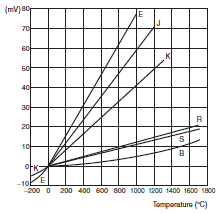If the thermocouple temperature sensor lead wire does not reach the temperature controller and the cable between the sensor and the temperature controller is extended with copper wire, a large temperature error will occur.
The thermocouple temperature sensor lead wires must be extended with compensating conductors.
A compensating conductor is a cable that produces nearly the same thermoelectromotive force as the thermocouple. There are general purpose cables (-20 to 90°C) and heat-resistant cables (0 to 150°C), depending on the ambient operating temperature. The characteristics of these cables are determined by JIS. Compensating conductors are available for each type of thermocouple. A compensating conductor that is suitable for the thermocouple must be used.

Platinum Resistance Thermometer

Resistance Thermometer

This device exploits the constant relation of metal resistanceto temperature.
Conditions requited for metal wire material:

(1) High temperature coefficient of electrical resistance and good linearity

(2) Stability

(3) Ability to be used with a broad temperature range

The material which best meets these conditions is platinum.
Only the platinum resistance thermometer is prescribed by JIS.

This device uses the characteristic of platinum (Pt) that causes its electrical resistance to increase in proportion to temperature.
Based on the 1989 revision of the JIS standards, platinum resistance thermometers that conformed to the previous standards were called JPt, and those that conformed to the 1989 and later standards were called Pt, but JPt was abolished with the 1997 revision. However, there are still systems in operation that use JPt, so temperature controllers also support JPt. The characteristics of Pt and JPt are different, therefore, the input type of the temperature controller must be correctly set.